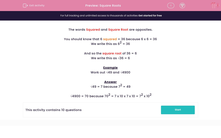# Square Roots

In this worksheet, students state the square roots of perfect squares.Key stage:  KS 2

Curriculum topic:   Maths and Numerical Reasoning

Curriculum subtopic:   Square Numbers

Difficulty level:#### Worksheet Overview

The words Squared and Square Root are opposites.

You should know that 6 squared = 36 because 6 x 6 = 36

We write this as 62 = 36

And so the square root of 36 = 6

We write this as √36 = 6

Example

Work out √49 and √4900

√49 = 7 because 72 = 49

√4900 = 70 because 70= 7 x 10 x 7 x 10 = 72 x 102

### What is EdPlace?

We're your National Curriculum aligned online education content provider helping each child succeed in English, maths and science from year 1 to GCSE. With an EdPlace account you’ll be able to track and measure progress, helping each child achieve their best. We build confidence and attainment by personalising each child’s learning at a level that suits them.

Get started